# HoTTSQL: Proving query rewrites with univalent SQL semantics

HoTTSQL: Proving query rewrites with univalent SQL semantics Chu et al., PLDI’17

Query rewriting is a vital part of SQL query optimisation, in which rewrite rules are applied to a query to transform it into forms with (hopefully!) a lower execution cost. Clearly when a query is rewritten we still want it to mean the same thing as the original – we call this semantic preserving. If you take Q1 and turn it into Q2, then for all database schemas and table instances Q1 and Q2 need to return the same results. Since query rewriting is used extensively in SQL engines, it may come as a surprise to you to learn that we don’t actually have proofs that many of the common rewrite rules actually are semantic preserving! Until now.

… while various rewrite rules have been proposed and studied extensively in the data management research community, to the best of our knowledge only some simple ones have been formally proven to be semantic preserving. This has unfortunately led to dire consequences as incorrect query results have been returned from widely-used database systems due to unsound rewrite rules, and such bugs can often go undetected for extended periods.

Consider the following query, which exposed a rewrite bug in Oracle 12c when the optimiser incorrectly converted the first left outer join in line 7 to a hash join.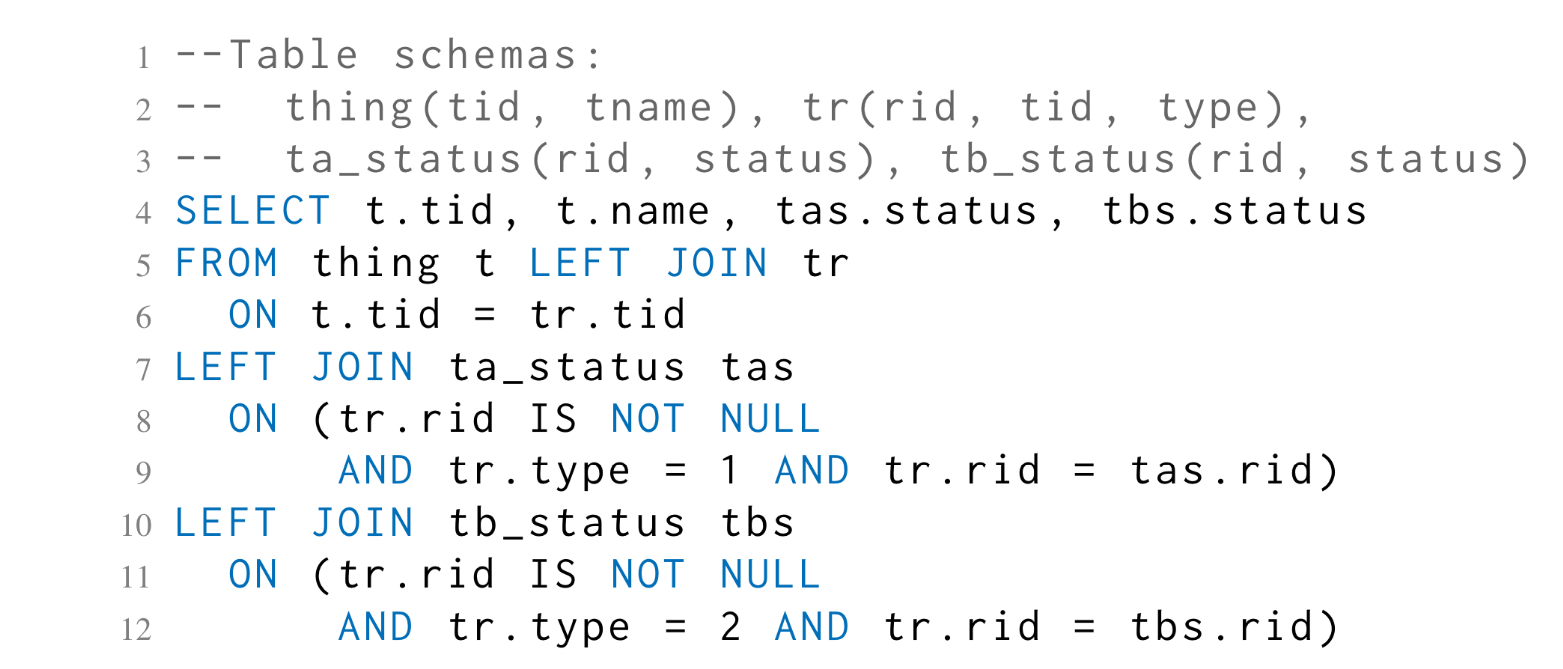HoTTSQL gives us a new SQL formalization that is suitable for proving the equivalence of queries, and on top of this a system to formally verify the equivalence of expressions. It’s all wrapped up in a simple to use interface, but as you might imagine, there’s a lot going on under the covers.

We demonstrate its utility by proving correct a large number of query rewrite rules that have been described in the literature and are currently used in popular database systems. We also show that, given counter examples, common mistakes made in query optimization fail to pass our formal verification, as they should.

This is a PLDI paper, so as you might expect it’s deep on the internals. For understanding HoTTSQL, I also highly recommend the Cosette website https://cosette.cs.washington.edu, and the higher-level CIDR’17 paper ‘Cosette: An automated prover for SQL.’

### The end-user perspective: proving the equivalence of rewrites

Cosette provides both a web interface and an API that permit you to describe schemas and tables, and queries over those tables, and then ask if they are equivalent. You express verification queries to Cosette using a simple DSL that looks like this: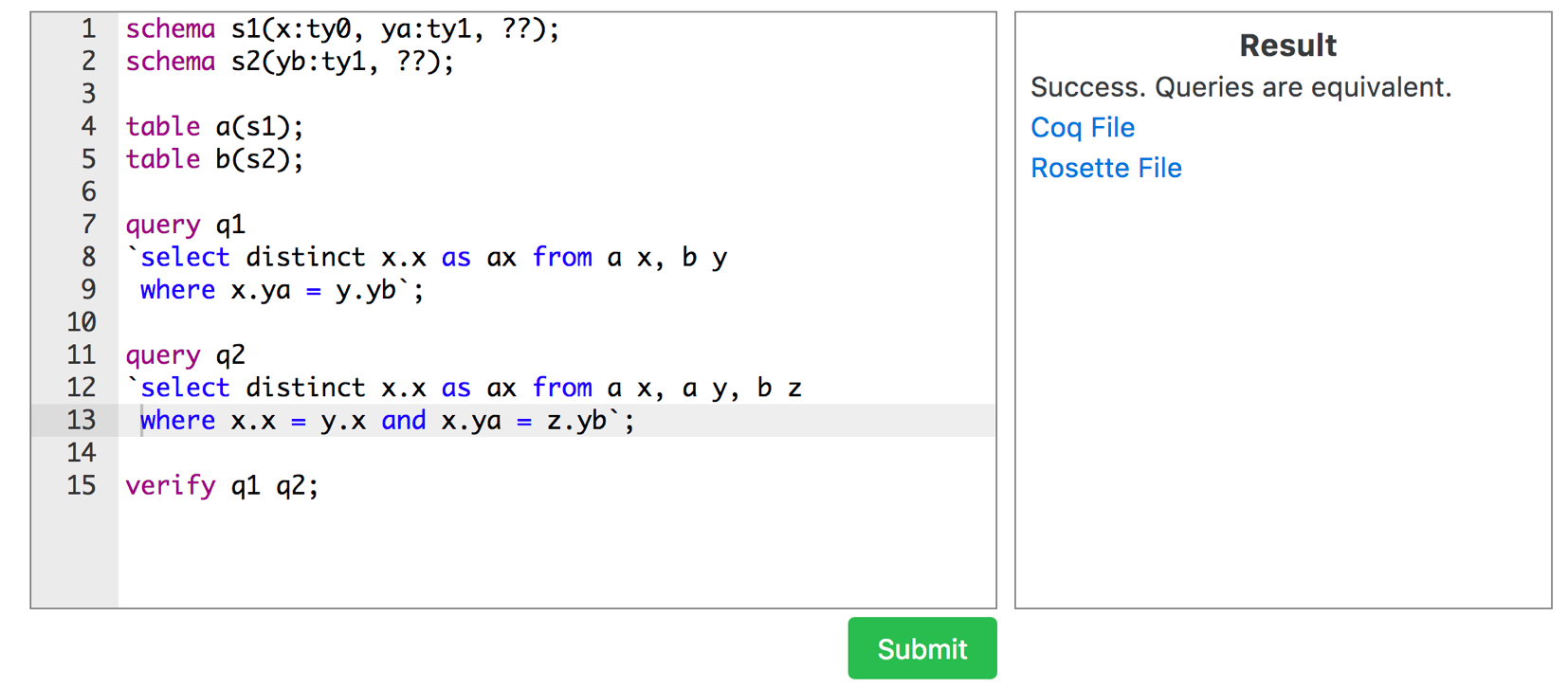You can try it out for yourself using the online hosted version here. The accompanying grammar looks like this, although you might find it easier to pick up from the Cosette Guide.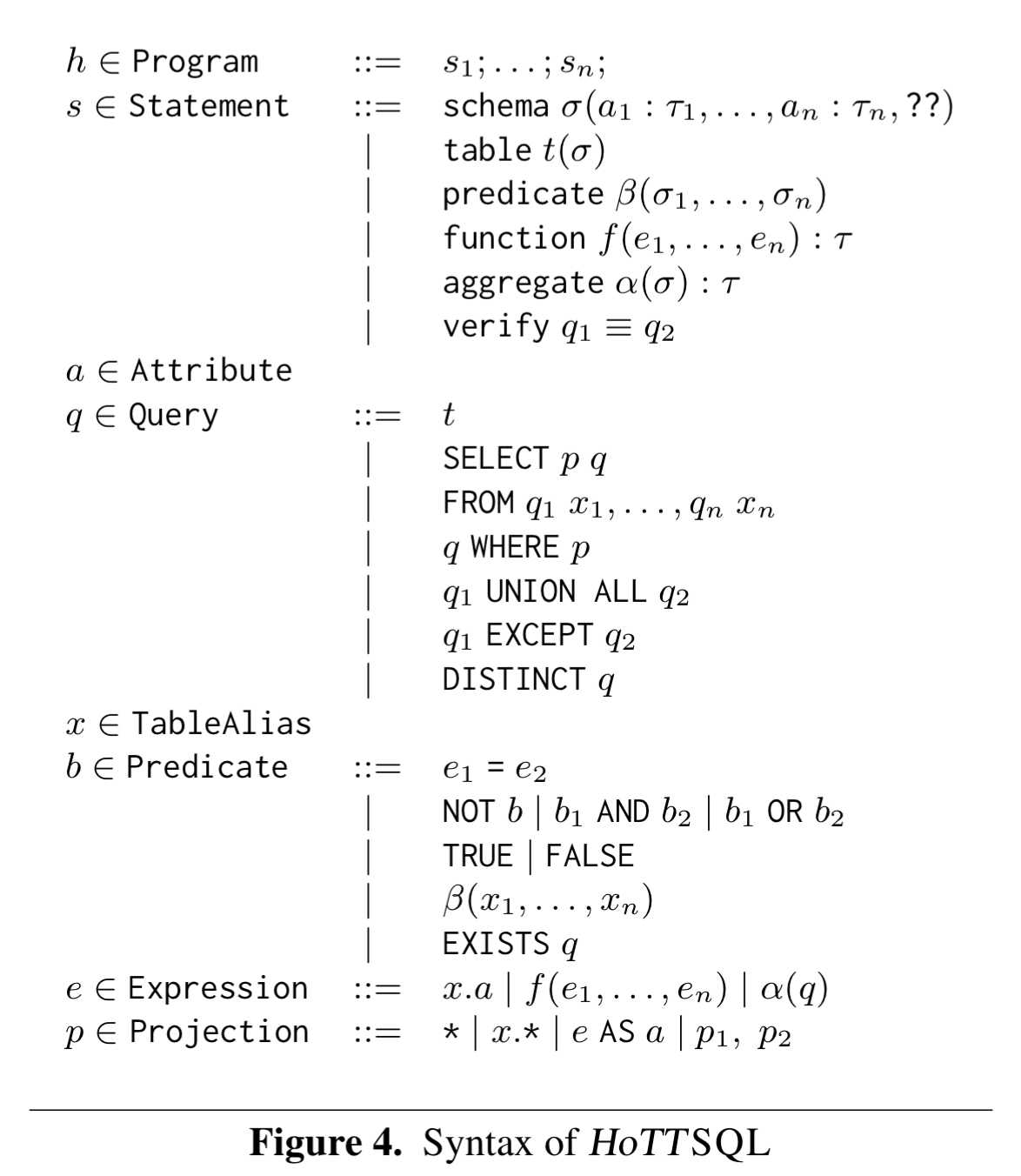Things work pretty much like you’d expect, but the use of wildcards is worth pointing out. A declaration such as:

[code lang=”text”]
schema s1(x: int, y:int, ??)
[/code]

Says that the schema must include at least an x column and a y column (both integer), but may have other columns as well although these aren’t used in the queries.

### Under the covers: SQL semantics

Under the covers there’s a lot going on to determine query equivalence. The big picture looks like this: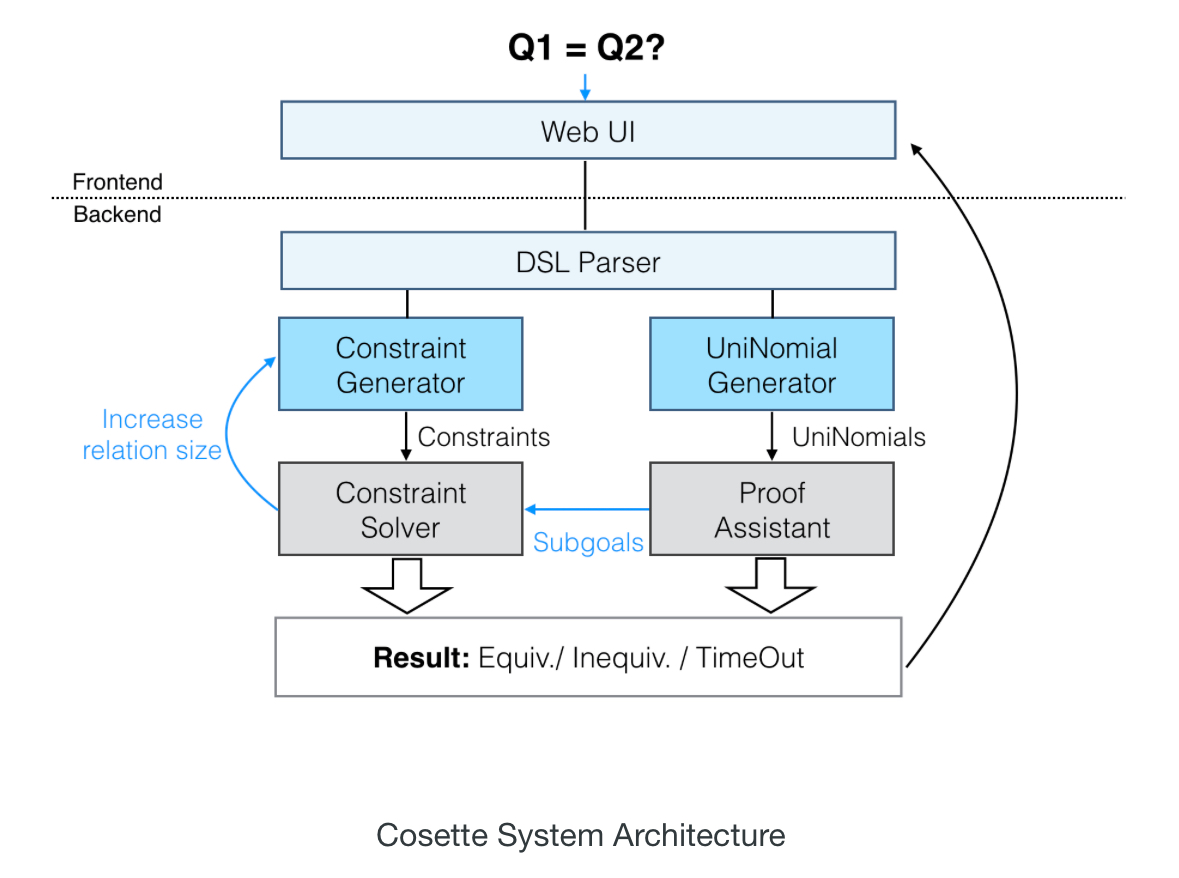A key part of the innovation is finding a suitable way to express the meaning of SQL queries (the fancy way of saying that is ‘denotational semantics’). The ANSI standard uses English prose known to be ambiguous in places. Previous attempts at formalisation used either a list-based semantics (which turned out to be very difficult to prove result equivalence with, due to all the permutations), or a set-based semantics (which is limited, because relations are actually bags!).

Our semantics consist of two non-trivial generalizations of K-relations, which represent a relation as a mathematical function that takes as input a tuple and returns its multiplicity, with 0 meaning that the tuple does not exist in the relation.

Because formal proving systems tend to be picky about the details (that’s their job!), K-relations as-is aren’t quite enough. Firstly we need to handle some subtleties around infinities, and secondly mapping tuples to simple counts turns out to be not quite enough. But if we replace the count numbers by univalent types and apply Homotopy Type Theory (HoTT) then we’re good to go again. The Coq proof assistant used by HoTTSQL has built in support for univalent types which simplified many of the proofs. To be honest though, unless you’re deep into your type theory, you can get an awful long way just by thinking of a mapping from tuples to simple numeric counts.

### K-relation SQL semantics – basics

When you think about it, there’s something special about the number zero when it comes to addition. It acts like an identity value, since$a + 0 = a$ and$0 + a = a$. For multiplication, the number one plays the same role:$a \times 1 = a$ and$1 \times a = a$. There’s another thing that addition and multiplication have in common, they’re both associative:$a \bullet(b \bullet c) = (a \bullet b) \bullet c$. This combination of a set and an associative binary option$\bullet$ defined over set members with a special identity value is sometimes useful to talk about in the abstract, so we give it a fancy name: a monoid. If we know that something is a monoid, then we already know that the following simple rewrite is valid:

•$a \bullet (b \bullet c) \equiv (a \bullet b) \bullet c$

A commutative monoid is one in which the binary operation commutes (i.e., the order of the operands doesn’t matter). So we can add one more simple rewrite to our list:

•$a \bullet b \equiv b \bullet a$

A set with both addition and multiplication monoid binary operations defined over its members is called a semi-ring. In a commutative semi-ring we know that both the addition and multiplication operations also commute. We also have the property that multiplication distributes over addition, giving a couple of extra rewrite rules:

•$(a + b) \times c \equiv (a \times c) + (b \times c)$
•$a \times (b + c) \equiv (a \times b) + (a \times c)$

We could describe such a semi-ring by a structure$\mathbf{K} = (K, + , \times, 0, 1)$. If we can find a way to express SQL semantics in terms of such a structure, then we can use all of the above rules to help us prove query rewrite equivalence. K-relations let us do just that. A K-relation is a function defined over some relation, written$[\![R]\!]$, that maps a tuple with given attribute values to a count of the number of times that tuple appears in the relation.

Given R as

Name Age
Alice 25
Alice 25
Bob 42

Then$[\![R]\!] (Alice,25) = 2$ and$[\![R]\!] (Bob, 42) = 1$.

• For a predicate b,$[\![b]\!] t$ is 1 is the predicate holds on the tuple, and 0 otherwise.
• For an attribute a, the projection$[\![a]\!] t$ returns the attribute a of the tuple t (not sure I like using the same bracket notation for all three purposes, but there you go…)
• We’ll also use a squash function, such that$|| x ||$ is 0 when x is 0, and 1 otherwise.
• And for equality,$(x = y)$ has value 0 when x is not equal to y, and 1 otherwise

We can now express all the relational operators in terms of these semi-ring operations: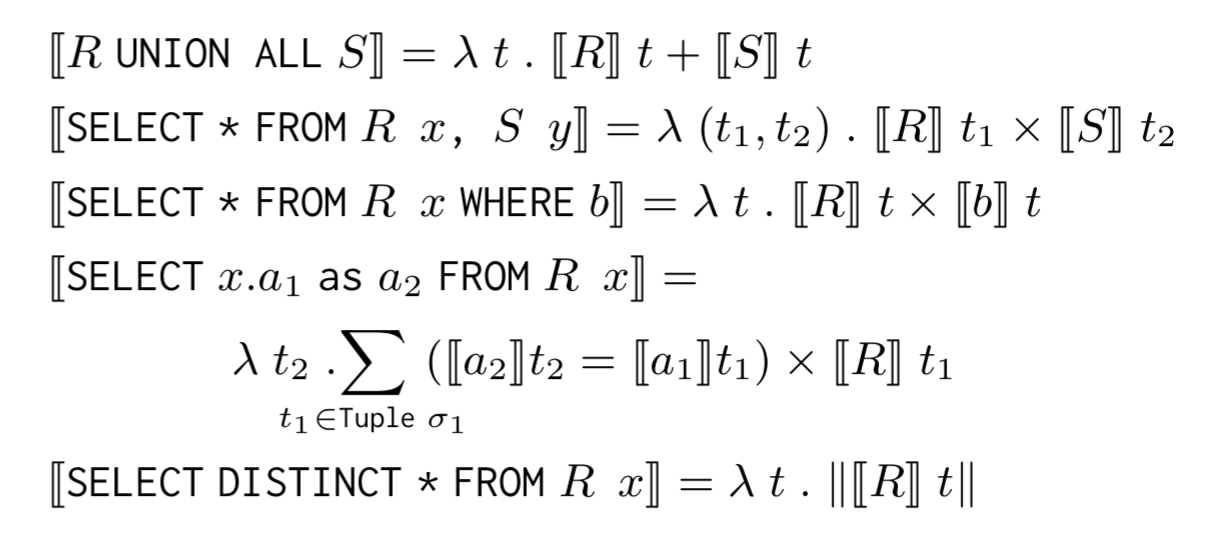K-Relation operators

And if you want to prove that two SQL queries are equal, you just have to prove that the two semi-ring expressions are equal. For example: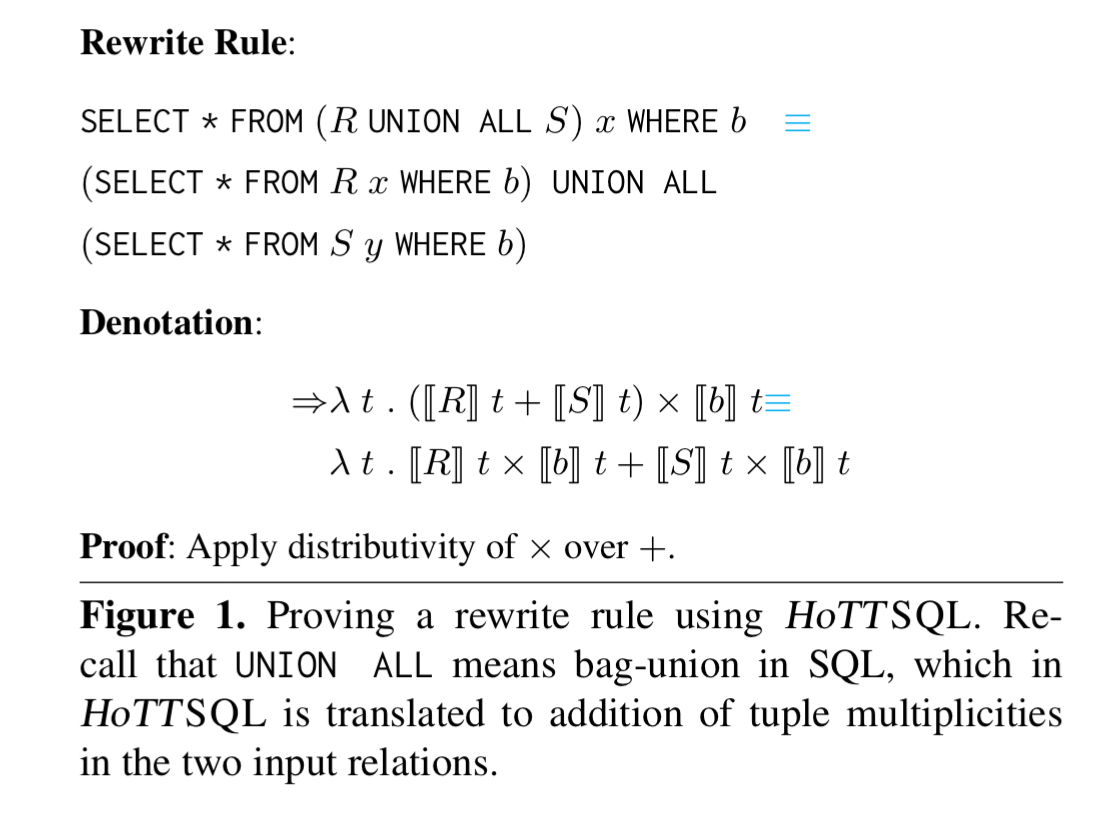### K-relation extensions

In K-relations as originally defined, the K-relation function needs ‘finite support.’ This means that the set of tuples with non-zero counts must be finite (relations don’t have infinite capacity):${ t | [\![R]\!] t \neq 0}$ is finite.

This creates a major problem for our equivalence proofs, since we need to prove, for each intermediate result of a SQL query, that it returns a relation with finite support. This adds significant complexity to the otherwise simple proofs of equivalence.

To get around this the authors do away with the requirement for relations to have finite support, and instead of mapping to simple counts, the relation function maps to the class of Homotopy types. “A homotopy type is an ordinary type with the ability to prove membership and equality between types.” These types generalise natural numbers and their semiring operations:

• The cardinal number 0 is the empty homotopy type 0
• The cardinal number 1 is the unit type 1
• Multiplication is the product type$\times$
• Addition is the sum type$+$
• Infinite summation is the dependent product type$\sum$
• Truncation is the squash type$|| \bullet ||$

All of this makes life easier in Coq, which already understands the rules for the Uninomial algebra, an algebra based on univalent types (homotopy types) with the above properties.

### Proving rewrite rules

The HoTTSQL DSL is translated to Uninomial form via an intermediate language HoTTIR. Section 4.3 in the paper describes the mapping. HoTTIR models schemas as collections of types organized in a binary tree, and tuples are dependent types on a schema.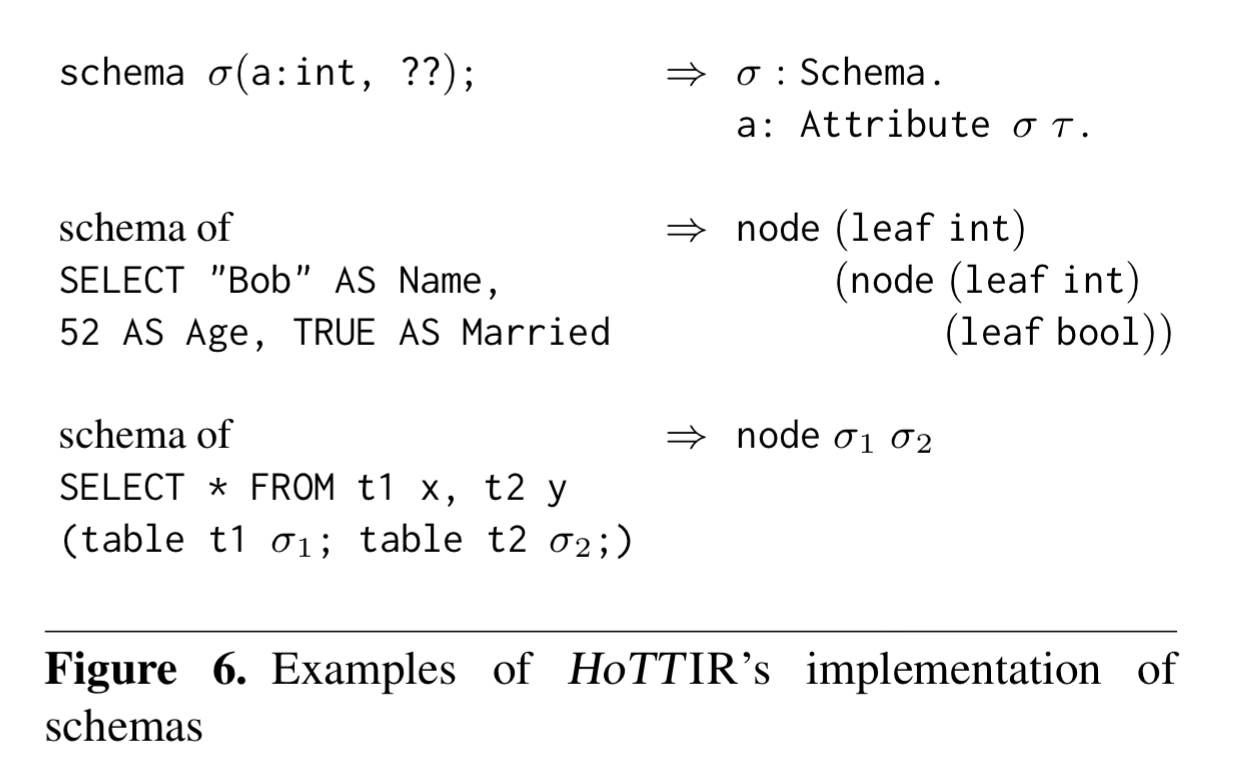We proved 23 rewrite rules from both the database literature and real world optimizers using HoTTSQL. Figure 9 (below) shows the number of rewrite rules that we proved in each category and the average lines of code (LOC) required per proof.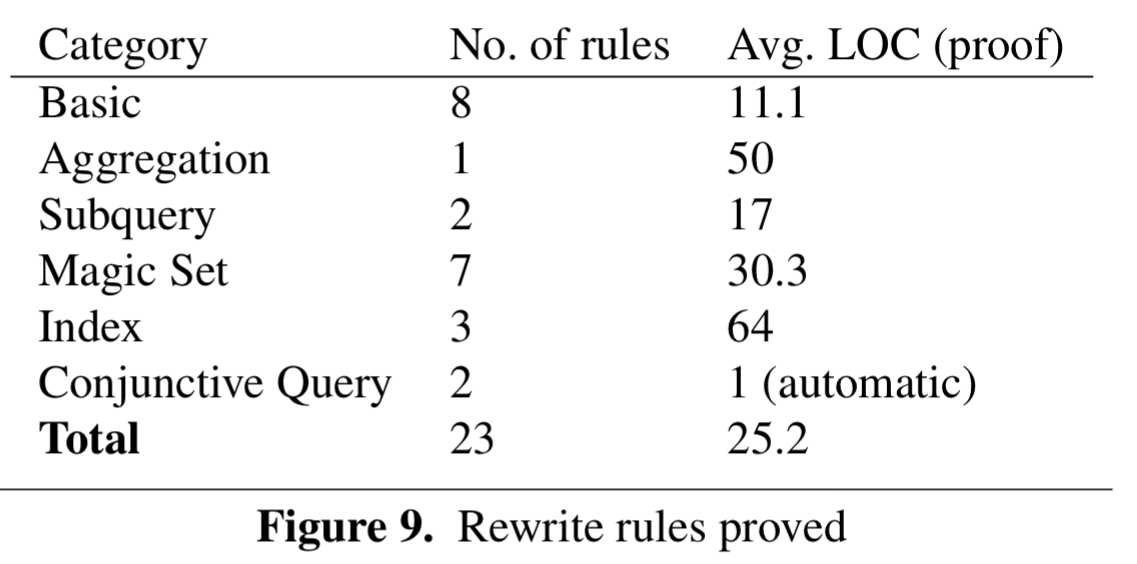For example, selection pushdown can be formulated as follows: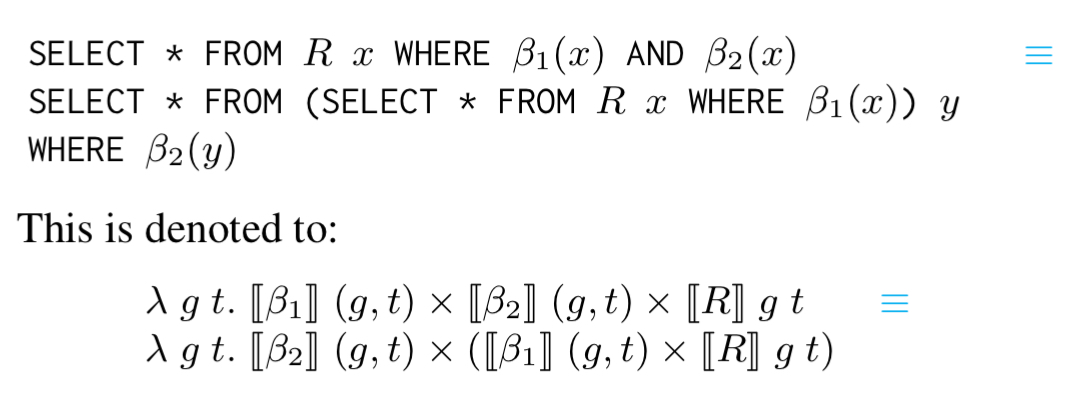“The proof proceeds by functional extensionality, along with the associativity and commutativity of$\times$.”